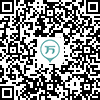140:002016年成人高考（专升本）《大学语文》真题
1～20小题，每小题2分，共40分。在每小题给出的四个选项中，只有一项是符合题目要求的，把所选项前的字母填在题后的括号内。
1
(单项选择题)

• A.

李商隐《无题》（相见时难别亦难）

• B.

李白《行路难》（其一）

• C.

陆游《关山月》（和戎诏下十五年）

• D.

杜甫《蜀相》

• A
• B
• C
• D

2
(单项选择题)

• A.

闻一多是新月社成员

• B.

《死水》是闻一多的代表作

• C.

闻一多诗歌的艺术风格是飘逸清丽

• D.

《发现》一诗抒发了闻一多的爱国主义激情

• A
• B
• C
• D

3
(单项选择题)

《陈情表》《张中丞传后叙》《报刘一丈书》的作者依次是（　）。

• A.

李密、宗臣、韩愈

• B.

宗臣、李密、韩愈

• C.

韩愈、李密、宗臣

• D.

李密、韩愈、宗臣

• A
• B
• C
• D

4
(单项选择题)

• A.

梁启超

• B.

胡适

• C.

鲁迅

• D.

陈独秀

• A
• B
• C
• D

5
(单项选择题)

“赋”作为一种文体，成熟于（　）。

• A.

战国时代

• B.

汉代

• C.

唐代

• D.

宋代

• A
• B
• C
• D

6
(单项选择题)

• A.

王实甫

• B.

马致远

• C.

自朴

• D.

关汉卿

• A
• B
• C
• D

7
(单项选择题)

• A.

《论语》

• B.

《战国策》

• C.

《史记》

• D.

《汉书》

• A
• B
• C
• D

8
(单项选择题)

“多行不义必自毙”“望洋兴叹”“形影相吊”三个成语依次出自（　）。

• A.

《郑伯克段于鄢》《庄子·秋水》《陈情表》

• B.

《庄子·秋水》《陈情表》《郑伯克段于鄢》

• C.

《郑伯克段于鄢》《陈情表》《庄子·秋水》

• D.

《陈情表》《庄子·秋水》《郑伯克段于鄢》

• A
• B
• C
• D

9
(单项选择题)

• A.

五四时期

• B.

抗日战争爆发初期

• C.

抗日战争胜利之时

• D.

新中国建立之时

• A
• B
• C
• D

10
(单项选择题)

“乐府”最初的含义是（　）。

• A.

先秦民歌

• B.

最早的格律诗

• C.

西汉王朝设立的掌管音乐的机构

• D.

宫廷里的歌舞厅

• A
• B
• C
• D

11
(单项选择题)

《五代史伶官传序》一文的主旨是（　）。

• A.

满招损，谦受益

• B.

祸患积于忽微

• C.

智勇多困于所溺

• D.

盛衰在于人事

• A
• B
• C
• D

12
(单项选择题)

• A.

《论语》

• B.

《孟子》

• C.

《庄子》

• D.

《墨子》

• A
• B
• C
• D

13
(单项选择题)

• A.

鲁迅——《坟》、《灯下漫笔》

• B.

朱自清——《背影》、《香市》

• C.

茅盾——《故都的秋》、《子夜》

• D.

钱钟书——《围城》、《论毅力》

• A
• B
• C
• D

14
(单项选择题)

《西厢记·长亭送别》中，“泪添九曲黄河溢，恨压三峰华岳低”两句采用的修辞手法是（　）。

• A.

对偶、夸张

• B.

比喻、通感

• C.

通感、夸张

• D.

比喻、排比

• A
• B
• C
• D

15
(单项选择题)

• A.

被后人称作“三苏”的作家是苏洵、苏轼、苏辙

• B.

“济南二安”指的是李清照和辛弃疾

• C.

苍凉悲壮是曹操诗歌的主要艺术风格

• D.

曹雪芹是我国明代伟大的浪漫主义作家

• A
• B
• C
• D

16
(单项选择题)

• A.

以子之道，移之官理，可乎

• B.

驱而之薛，使吏召诸民当偿者，悉来合券

• C.

故远人不服，则修文德以来之

• D.

不如早为之所，无使滋蔓

• A
• B
• C
• D

17
(单项选择题)

• A.

盘庚不为怨者故改其【度】；【度】义而后动,是而不见可悔故也

• B.

长铗【归】来乎！食无鱼；男有分，女有【归】

• C.

危而不持，颠而不扶，则将焉用彼【相】矣；孟尝君为【相】数十年，无纤介之祸者，冯谖之计也

• D.

使吏如诸民当偿【者】,悉来合券；广曰：“是必射雕【者】也”

• A
• B
• C
• D

18
(单项选择题)

• A.

都城过百雉，国之害也。

• B.

树之以桑，五十者可以衣帛矣。

• C.

不拊爱子其民，因而贾利之。

• D.

过其友曰：“孟尝君客我！”

• A
• B
• C
• D

19
(单项选择题)

• A.

青海长云暗雪山 暗：使……暗

• B.

诚既勇兮又以武，终刚强兮不可凌 诚：果然

• C.

女之耽兮，不可说也 说：述说

• D.

吾非至于子之门则殆矣 殆：危险

• A
• B
• C
• D

20
(单项选择题)

• A.

生孩六月，慈父见背；行年四岁，舅夺母志。

生孩六月，慈父见背：生下来才六个月，慈父就去世了

• B.

今乃弃黔首以资敌国，却宾客以业诸侯。

弃黔首以资敌国：舍弃了黔的首领去资助敌对的国家

• C.

国不堪贰，君将若之何？

国不堪贰：国家不能使土地有两属的情况存在

• D.

两家子弟材智下，不能通知二父志。

不能通知二父志：不能理解他们父亲的志向

• A
• B
• C
• D